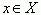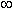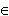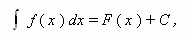# Primitive. Indefinite integral

Primitive. Finding of primitive: infinite set of solutions.
Indefinite integral. Constant of integration.

Primitive. A continuous function F ( x ) is called a primitive for a function f ( x ) on a segment X ,  if for eachF’ ( x ) = f ( x ).

E x a m p l e . The function F ( x ) = x 3 is a primitive for the function f ( x ) = 3 x 2 on the
interval  ( -, +) , because

F’ ( x ) = ( x 3 ) = 3 x 2 = f ( x )

for all x( -, +) .
It is easy to check, that  the function x 3 + 13  has the same derivative 3 x 2 ,
so it is also a primitive for the function
3 x 2 for all x( -, +) .

It is clear, that instead of 13 we can use any constant. Thus, the problem of finding a primitive has an infinite set of solutions. This fact is reflected in the definition of an indefinite integral:

Indefinite integral of a function f ( x ) on a segment X is a set of all its primitives . This is written as :where C – any constant, called a constant of integration .